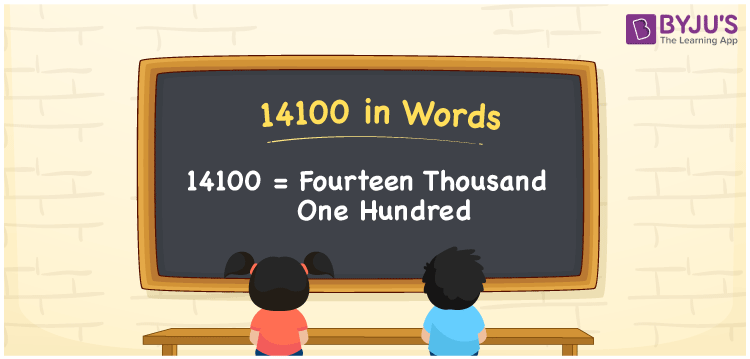# 14100 in words

14100 in words is written as Fourteen Thousand One Hundred. In 14100, 1 has a place value of ten thousand and 4 has a place value of thousand and 1 has a place value of hundred. The article on Place Value gives more information. The number 14100 is used in expressions that relate to money, distance, length, count and many more. For example, “The Note on a book read that it has Fourteen Thousand One Hundred words in it.”

 14100 in words Fourteen Thousand One Hundred Fourteen Thousand One Hundred in Numbers 14100

## 14100 in English Words## How to Write 14100 in Words?

We can convert 14100 to words using a place value chart. The number 14100 has 5 digits, so let’s make a chart that shows the place value up to 5 digits.

 Ten thousand Thousands Hundreds Tens Ones 1 4 1 0 0

Thus, we can write the expanded form as:

1 × Ten thousand + 4 × Thousand + 1 × Hundred + 0 × Ten + 0 × One

= 1 × 10000 + 4 × 1000 + 1 × 100 + 0 × 10 + 0 × 1

= 14100

= Fourteen Thousand One Hundred.

14100 is the natural number that is succeeded by 14099 and preceded by 14101.

14100 in words – Fourteen Thousand One Hundred.

Is 14100 an odd number? – No.

Is 14100 an even number? – Yes

Is 14100 a perfect square number? – No

Is 14100 a perfect cube number? – No

Is 14100 a prime number? – No

Is 14100 a composite number? – Yes

## Solved Example

1. Write the number 14100 in expanded form

Solution: 1 x 10000 + 4 x 1000 + 1 x 100 + 0 x 10 + 0 x 1

Or Just 1 x 10000 + 4 x 1000 + 1 x 100

We can write 14100 =10000 + 4000 + 100 + 0 + 0

= 1 x 10000 + 4 x 1000 + 1 x 100 + 0 x 10 + 0 x 1

## Frequently Asked Questions on 14100 in words

Q1

### How to write 14100 in words?

14100 in words is written as Fourteen Thousand One Hundred.
Q2

### State True or False. 14100 is divisible by 3?

True. 14100 is divisible by 3.
Q3

### Is 14100 divisible by 10?

Yes. 14100 is divisible by 10.